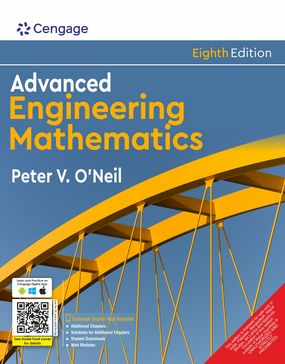###### Home
>

Higher EducationAuthor(s): Peter V. O'Neil

ISBN: 9789355739964

Edition: 8th

#### Rs. 825

Binding: Paperback

Pages: 868

Trim Size : 254 x 203 mm

ADVANCED ENGINEERING MATHEMATICS, 8E is written specifically for students like you, who are primarily interested in how to effectively apply mathematical techniques to solve advanced engineering problems. Numerous examples include illustrations of mathematical techniques as well as applications. A "Math in Context" feature clearly demonstrates how the mathematical concepts and methods you are learning relate to current engineering problems. The book is organized into seven distinctive parts to provide precise focus on individual mathematical ideas and methods. A student solutions manual offers detailed solutions to half of the problems in the book for your use in checking your progress as well as study and review.

• Detailed examples highlight both mathematical ideas and their applications. Valuable examples throughout develop and emphasize mathematical concepts as well as practical engineering uses.
• A guide to post-calculus notation assists students. This helpful guide provides support for your students as they reference new terms and symbols.
• The book’s seven-part organization best fits most course organizations. Seven distinctive parts clearly focus and differentiate the mathematical ideas and methods while giving you the flexibility to select the sections best suited for your course and student needs.
• An instructor’s solutions manual offers complete solutions. You will find detailed solutions to virtually all problems in the book to save you time and effort.
• Two additional web chapters detail statistics and probability. These chapters are conveniently posted on the book’s website and include both problems and solutions.
• Tables of transforms provide quick reference. Your students will find tables for Fourier, Laplace, Fourier cosine and sine for use in solving problems within the book.
• Detailed examples illustrate the use of notation and the theory. The numerous examples clarify notation, theory, and the underlying computations, followed by the numerical calculations themselves.
• Student solutions manual contains worked-out solutions to half of the text problems. These solutions are specifically developed to assist your students in truly understanding the ideas involved, rather than simply listing the mechanical steps.

PART I: ORDINARY DIFFERENTIAL EQUATIONS

1. First-Order Differential Equations.

2. Second-Order Differential Equations.

3. The Laplace Transform.

4. Eigenfunction Expansions.

Part II: PARTIAL DIFFERENTIAL EQUATIONS

5. The Heat Equation.

6. The Wave Equation.

7. Laplace’s Equation.

8. Special Functions and Applications.

9. Transform Methods of Solution.

Part III: MATRICES AND LINEAR ALGEBRA

10. Vectors and the Vector Space Rn.

11. Matrices, Determinants and Linear Systems.

12. Eigenvalues, Diagonalization and Special Matrices.

PART IV: SYSTEMS OF DIFFERENTIAL EQUATIONS

13. Systems of Linear Differential Equations.

14. Nonlinear Systems and Qualitative Analysis.

PART V: VECTOR ANALYSIS

15. Vector Differential Calculus.

16. Vector Integral Calculus.

PART VI: FOURIER ANALYSIS

17. Fourier Series.

18. Fourier Transforms.

PART VII: COMPLEX FUNCTIONS

19. Complex Numbers and Functions.

20. Integration.

21. Series Representations of Functions.

22. Singularities and the Residue Theorem.

23. Conformal Mappings.

Dr. Peter O’Neil

Dr. Peter O’Neil has been a professor of mathematics at the University of Alabama at Birmingham since 1978. At the University of Alabama at Birmingham, he has served as chairman of mathematics, dean of natural sciences and mathematics, and university provost. Dr. Peter O’Neil has also served on the faculty at the University of Minnesota and the College of William and Mary in Virginia, where he was chairman of mathematics. He has been awarded the Lester R. Ford Award from the Mathematical Association of America. He received both his M.S and Ph.D. in mathematics from Rensselaer Polytechnic Institute. His primary research interests are in graph theory and combinatorial analysis.